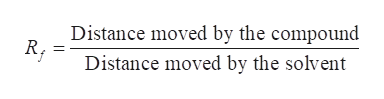Suppose the distance a compund moved in a paper chromatography experiment was 30 millameters and the solvent front was 61 millameters. What would the Rf (retention factor) be, and why?

Question

Suppose the distance a compund moved in a paper chromatography experiment was 30 millameters and the solvent front was 61 millameters. What would the Rf (retention factor) be, and why?

Step 1

We have to calculate the Rf value when in paper chromatography, the distance moved by the compound was 30 millimetres and the solvent front was 61 millimetres.

Step 2

In paper chromatography, the retention factor of any substance in the mixture is the ratio of the distance moved by the substance above the origin to the distance moved by the solvent.help_outlineImage TranscriptioncloseDistance moved by the compound Distance moved by the solvent fullscreen
Step 3

Distance moved by the compound = 30 millimetre

Dista...

Want to see the full answer?

See Solution

Want to see this answer and more?

Our solutions are written by experts, many with advanced degrees, and available 24/7

See Solution
Tagged in

General Chemistry